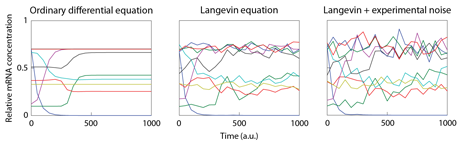## Java library for simulating stochastic differential equations

libSDE is an open-source Java library for simulating systems of stochastic differential equations (SDEs). SDEs are used to model diverse phenomena such as white noise and Brownian motion (also called Wiener process). Application domains include financial mathematics and economics, signal processing, chemistry, quantum mechanics, meteorology and music.

libSDE implements:

• Itô and Stratonovich schemes
• Euler-Maruyama, Euler-Heun, derivative-free Milstein and Stochastic Runge-Kutta (SRK15) solvers

A brief introduction to the simulation of systems of SDEs is given in Numerical Integration of SDE: A Short Tutorial.

libSDE has been developed during my PhD thesis. T Schaffter, From genes to organisms: Bioinformatics System Models and Software, 2014.

## Simulating molecular noise in the dynamics of gene networks

In living cells, molecular noise originates from thermal fluctuations and noisy processes such as transcription and translation. Hence, random fluctuations affect concentration levels of mRNA and protein, whose expression can be viewed as a stochastic process.

The model of molecular noise that we have developed uses Langevin equations. We have implemented this model in GeneNetWeaver (GNW) to generate data sets for assessing the performance of network inference methods in the fourth and fifth editions of the DREAM Project, an annual community-wide network inference challenge.The integration of the ODE model of gene regulation in GNW leads to noiseless gene expression. If molecular noise is selected, the ODE model is replaced by a system of stochastic differential equations (SDEs) called Langevin equations which is described here.

The measurement noise depends on the technology used to monitor gene expression concentrations and is modeled independently of the molecular noise. GNW implements Gaussian and log-normal models of experimental noise as well as a model of noise observed in microarrays.

## Other SDE libraries

• SDE Toolbox: simulation and estimation of stochastic differential equations with Matlab.
• SDE library for R: simulation and inference for stochastic differential equations.
• SDE by Desmond Higham: SDE is a C library which illustrates the properties of stochastic differential equations and some algorithms for handling them.
• libMoM: library for stochastic simulations in engineering using method of moments (libMoM). The library is written in C++. The user interface programs are in C.

Do you know another one?